This paper is built upon Elastic Weight Consolidation 1 (EWC), a common method to avoid catastrophic forgetting. In this setting, we want to learn the $$Kth$$ task without forgetting the $$K-1$$ previous tasks. To do so, EWC compute the optimal $$\theta_{:K}$$ given $$\theta_{:K-1}$$ such that it maximizes the posterior $$p_{:K} = p(\theta \vert D_{:K})$$ where $$D_{:K}$$ are all datasets up to task $$K$$.

This can be done using the Fisher matrix of previous task $$\bar F_{:K-1}$$ (See eq. 3).

An assumption that EWC requires is that the Fisher matrix must be block diagonal, which is hard to get in practice.

This paper aims to approximate a block diagonal Fisher matrix by doing a reparameterization of all weights in the network. I’ll only describe the process for the fully-connected layer, but the process is similar for CNNs.

Using eq. 7, we can approximate the Fisher matrix from two components which are available : the inputs and the gradients.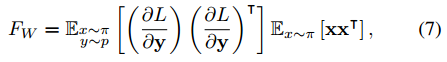From those two components, we can find two new weights $$U_{1}, U_{2}$$. These weights will provide an approximate diagonalisation of $$W$$, thus preventing catastrophic forgetting. The learnable weight then become $$W' = U^{T}_{2}WU^{T}_{1}$$.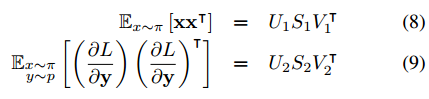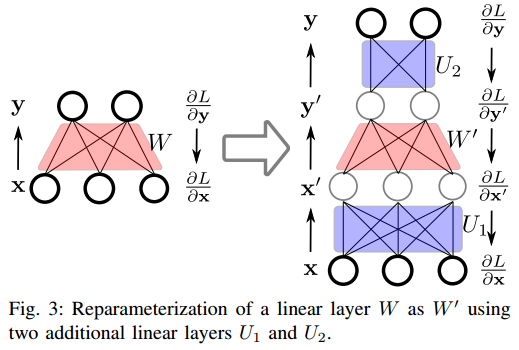## Results

On multiple datasets, the authors show that their method works better than standard EWC.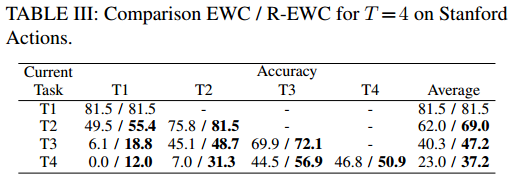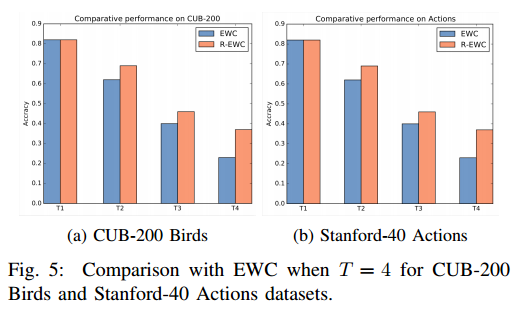1. Kirkpatrick, J., Pascanu, R., Rabinowitz, N., Veness, J., Desjardins, G., Rusu, A. A., … & Hassabis, D. (2017). Overcoming catastrophic forgetting in neural networks. Proceedings of the National Academy of Sciences, 114(13), 3521-3526.